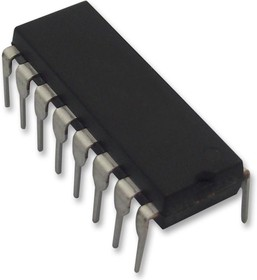Мой регион: Россия
Единая справочная
+7 495 544-00-08

# CD4527BE

Ном. номер: 9000424451
Производитель: Texas Instruments150 руб. 52 шт. со склада г.Москва,срок 2-3 недели от 3 шт. — 90 руб. от 10 шт. — 68 руб.
 Добавить в корзину 1 шт. на сумму 150 руб.

The CD4527BE is a low-power 4-bit Digital Rate Multiplier that provides an output-pulse rate which is the clock-input-pulse rate multiplied by 1/10 times the BCD input. For example, when the BCD input is 8, there will be 8 output pulses for every 10 input pulses. This device may be used to perform arithmetic operations (add, subtract, divide, raise to a power), solve algebraic and differential equations, generate natural logarithms and trigonometric functions, A/D and D/A conversion and frequency division. For fractional multipliers with more than one digit, CD4527B devices may be cascaded in two different modes: the Add mode and the Multiply mode.

• Cascadeable in multiples of 4-bit
• Set to 9 input and 9 detect output
• 100% Test for quiescent current at 20V
• Maximum input current of 1µA at 18V Over full package-temperature range;
• 2.5V at VDD=15V Noise margin (full package-temperature range)
• Meets all requirements of JEDEC Tentative Standard No. 13A

Выберите регион, чтобы увидеть способы получения товара.